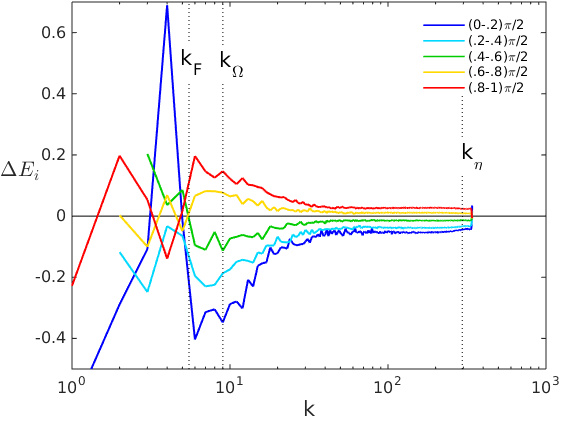# Laboratoire de Mécanique des Fluides et d’Acoustique - UMR 5509

LMFA - UMR 5509
Laboratoire de Mécanique des Fluides et d’Acoustique
Lyon
France

Donato Vallefuoco

## Concurrent spectral and separation-space views of small-scale anisotropy in rotating turbulence

14 mars 2017 13h ECL bât I11 salle de réunion 1er étage

Rotating turbulence is central in many contexts, e.g. astrophysical, geophysical and industrial flows. A background rotation about a fixed axis introduces anisotropy in the turbulent dynamics through both linear and nonlinear mechanisms. The flow regime can be characterized by two independent non-dimensional parameters, e.g. the Reynolds and the Rossby numbers or, equivalently, the ratio of the integral scale to the Kolmogorov scale $L/\eta$, and the ratio $l_\Omega/L$, where $l_\Omega=\sqrt{\epsilon/(2\Omega)^3}$ is referred to as the Zeman scale, $\epsilon$ is the mean dissipation and $\Omega$ is the rotation rate. The Zeman scale is the scale $l$ at which the inertial timescale $(l^2/\epsilon)^{1/3}$ equals the rotation timescale $1/\Omega$, and thus, if the Reynolds number is large, scales much larger than $l_\Omega$ are mainly affected by rotation while scales much smaller than $l_\Omega$ are dominated by the nonlinear dynamics and are expected to recover isotropy.

High Reynolds number forced DNS of non-helical and helical incompressible rotating turbulence in a periodic box are performed. We characterize scale-dependent anisotropy through (a) energy direction-dependent spectra in Fourier space, and (b) distributions of the third-order two-point vector moment in the separation space $\boldsymbol{F}=$, where $d\boldsymbol{u}=\boldsymbol{u}(\boldsymbol{x}+\boldsymbol{r})-\boldsymbol{u}(\boldsymbol{x})$ and $\boldsymbol{r}$ is the separation vector.

We focus on the low-rotation regime, where the Zeman scale is larger than the Kolmogorov scale. We show that a high-anisotropy small-wavenumber range and a low-anisotropy large-wavenumber range arise. In the former range anisotropy quickly decreases with wavenumber while in the latter range anisotropy slightly increases with wavenumber. Importantly, anisotropy arises even at scales much smaller than the Zeman scale and no isotropy recovery is observed at small scales, in contrast with the above classical dimensional arguments.
Next, we estimate the value of the threshold wavenumber between large-anisotropy wavenumbers and low-anisotropy wavenumbers, and show that it depends on both the Zeman scale and the micro-Rossby number $\omega'/(2\Omega)$, where $\omega'$ is the r.m.s. of vorticity.

Finally, anisotropy at scales much smaller than the Zeman scale can be detected in separation space too. In particular, we find that the radial component of $\boldsymbol{F}$ is negative and increases in modulus with the polar angle (between $\boldsymbol{r}$ and $\boldsymbol{\Omega}$), its polar component is negative, and its azimuthal component is positive.
However, we show that small-scale anisotropy in separation space is qualitatively different from large-scale anisotropy, which conforms with the inertial theory.